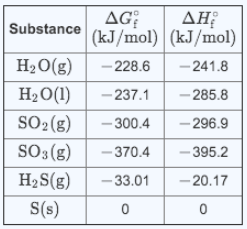# Problem: The reactionSO2(g) + 2H2S(g) ⇋ 3S (s) + 2H2O(g) is the basis of a suggested method for removal of SO2, a pollutant that irritates airways causing coughing, from power-plant stack gases.The values below may be helpful when answering questions about the process.Calculate the equilibrium constant Kp for the reaction at a temperature of 298 K.

###### FREE Expert Solution

We’re being asked to determine the equilibrium constant (K) at 298 K for the given reaction:

SO2(g) + 2 H2S(g) ⇋ 3 S(s) + 2H2O(g)

Step 1: Solve for Δrxn:

81% (429 ratings)###### Problem Details

The reaction

SO2(g) + 2H2S(g) ⇋ 3S (s) + 2H2O(g)

is the basis of a suggested method for removal of SO2, a pollutant that irritates airways causing coughing, from power-plant stack gases.

The values below may be helpful when answering questions about the process.Calculate the equilibrium constant Kp for the reaction at a temperature of 298 K.

Frequently Asked Questions

What scientific concept do you need to know in order to solve this problem?

Our tutors have indicated that to solve this problem you will need to apply the Gibbs Free Energy concept. You can view video lessons to learn Gibbs Free Energy. Or if you need more Gibbs Free Energy practice, you can also practice Gibbs Free Energy practice problems.